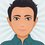# How can I substitute here?

I don't understand how to find the answer to this. Any help will be appreciated!

What is the value of $$x$$ if

$x= \sqrt{1+2\sqrt{1+4\sqrt{1+16\sqrt{1+256\sqrt{1+\cdots}}}}}$? ​Note by Vinayak Srivastava
1 month, 3 weeks ago

This discussion board is a place to discuss our Daily Challenges and the math and science related to those challenges. Explanations are more than just a solution — they should explain the steps and thinking strategies that you used to obtain the solution. Comments should further the discussion of math and science.

When posting on Brilliant:

• Use the emojis to react to an explanation, whether you're congratulating a job well done , or just really confused .
• Ask specific questions about the challenge or the steps in somebody's explanation. Well-posed questions can add a lot to the discussion, but posting "I don't understand!" doesn't help anyone.
• Try to contribute something new to the discussion, whether it is an extension, generalization or other idea related to the challenge.

MarkdownAppears as
*italics* or _italics_ italics
**bold** or __bold__ bold
- bulleted- list
• bulleted
• list
1. numbered2. list
1. numbered
2. list
Note: you must add a full line of space before and after lists for them to show up correctly
paragraph 1paragraph 2

paragraph 1

paragraph 2

[example link](https://brilliant.org)example link
> This is a quote
This is a quote
    # I indented these lines
# 4 spaces, and now they show
# up as a code block.

print "hello world"
# I indented these lines
# 4 spaces, and now they show
# up as a code block.

print "hello world"
MathAppears as
Remember to wrap math in $$ ... $$ or $ ... $ to ensure proper formatting.
2 \times 3 $2 \times 3$
2^{34} $2^{34}$
a_{i-1} $a_{i-1}$
\frac{2}{3} $\frac{2}{3}$
\sqrt{2} $\sqrt{2}$
\sum_{i=1}^3 $\sum_{i=1}^3$
\sin \theta $\sin \theta$
\boxed{123} $\boxed{123}$

Sort by:

$\sqrt{1+2\sqrt{1+4\sqrt{1+8\sqrt{1+16\sqrt{\cdots}}}}}$ or $\sqrt{1+2\sqrt{1+4\sqrt{1+16\sqrt{1+256\sqrt{\cdots}}}}}$?

- 1 month, 1 week ago

Vinayak asked for the second expression - which diverges. The first expression is also very interesting, I haven't found the solution to that yet, just estimated the result to be around 4.14031456214126 using python (look at the comments under my solution). If you find the exact result for the first expression, please let me know!

- 1 month, 1 week ago

- 1 month, 1 week ago

I think that the expression does not converge at all. To show that, we will proof $n\sqrt{1 + n^2\sqrt{1 + n^4\sqrt{...}}} > n^k$ for all natural numbers $n$ and $k$. Here is the proof by induction on $k$:

START: k = 1 The values of all square roots in the expression are greater than 1, so we have: $n\sqrt{1 + ... } > n = n^1$

STEP from k to k +1:

We assume $n\sqrt{1 + n^2\sqrt{1 + n^4\sqrt{...}}} > 2^k$ for all $n$ and a particular $k$.

This also applies to $n^2$, so we have: $n^2\sqrt{1 + n^4\sqrt{...}} > (n^2)^k = n^{2k}$

We conclude: $n\sqrt{1 + n^2\sqrt{1 + n^4\sqrt{...}}} > n\sqrt{1 + n^{2k}} > n\sqrt{n^{2k}} = n \times n^k = n^{k+1}$

The start of induction and the step of induction show the assumption for all $k$.

We can apply this statement for $n = 2$:

$2\sqrt{1 + 4\sqrt{1 + 16\sqrt{...}}} > 2^k$

This will exceed any real number for large $k$ and does not converge. Therefore, the whole expression does not converge, the value of $x$ is undefined.

- 1 month, 3 weeks ago

Thanks a lot @Finnley Paolella! Just asking of curiosity, do you know of any expressions like this(I mean continuing infinitely0), which look like they won't converge, but they do?

- 1 month, 2 weeks ago

I think the expression $\sqrt{1 + 2\sqrt{1 + 4\sqrt{1 + 8\sqrt{1 + 16\sqrt{1 + \dots}}}}}$ converges.

- 1 month, 2 weeks ago

How can I find the value of this expression?

- 1 month, 2 weeks ago

According to this code, it is around 4.14031...

 1 2 3 4 5 6 7 8 9 import math def f(n, depth): if depth == 0: return 1 return math.sqrt(1 + n * f(2*n, depth - 1)) print(f(2, 5)) print(f(2, 50)) print(f(2, 200)) print(f(2, 500)) 

3.6136728719051603

4.140314562141137

4.14031456214126

4.14031456214126

- 1 month, 2 weeks ago

Thank you very much! Any algebraic method?

- 1 month, 2 weeks ago

I am sure there is... but I haven't found one.

- 1 month, 2 weeks ago

OK, no problem, thanks for spending your time on my question!

- 1 month, 2 weeks ago